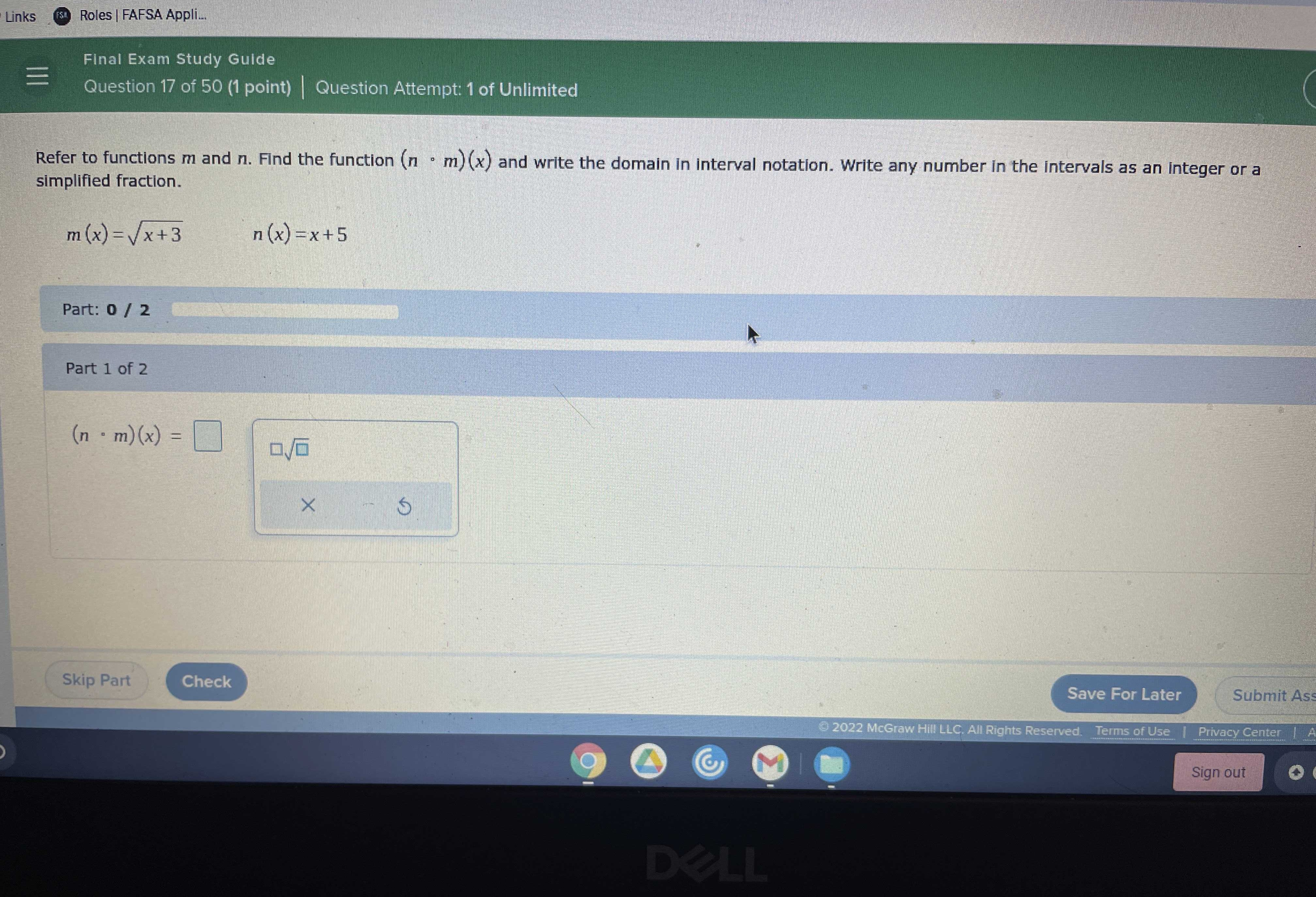### Still have math questions?

Algebra
Question

Refer to functions $$m$$ and $$n$$ . Find the function $$( n \cdot m ) ( x )$$ and write the domain in interval notation. Write any number in the intervals as an integer or a simplified fraction.

$$m ( x ) = \sqrt { x + 3 } n ( x ) = x + 5$$

$$( n \cdot m ) ( x ) = \square$$And what’s the domain

$$(n \cdot m)(x) = \sqrt{x+ 3}+ 5$$

Domain:

Interval Notation: $$[−3,∞)$$

Set-Builder Notation: $$\{x|x≥−3\}$$

Solution
View full explanation on CameraMath App.